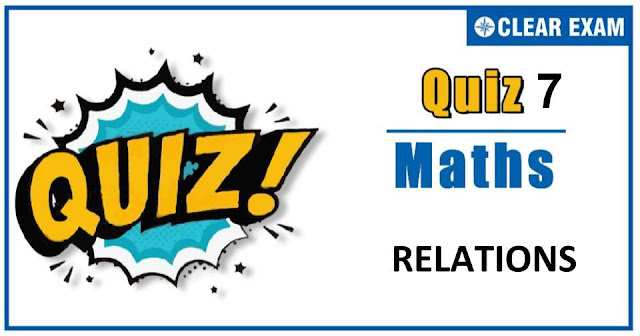## MATHEMATICS RELATIONS QUIZ-7

As per analysis for previous years, it has been observed that students preparing for JEE MAINS find Mathematics out of all the sections to be complex to handle and the majority of them are not able to comprehend the reason behind it. This problem arises especially because these aspirants appearing for the examination are more inclined to have a keen interest in Mathematics due to their ENGINEERING background.

Furthermore, sections such as Mathematics are dominantly based on theories, laws, numerical in comparison to a section of Engineering which is more of fact-based, Physics, and includes substantial explanations. By using the table given below, you easily and directly access to the topics and respective links of MCQs. Moreover, to make learning smooth and efficient, all the questions come with their supportive solutions to make utilization of time even more productive. Students will be covered for all their studies as the topics are available from basics to even the most advanced.

Q3.
•  -√3≤x≤+√3
•  -1-√3≤x≤-1+√3
•  -2≤x≤2
•  -2+√3≤x≤-2-√3
Solution
For domain of f(x),2-2x-x2≥0 ⇒ x2+2x-2≤0 ⇒ -1-√3≤x<-1 span="">

Q4. Let f be a real valued function with domain R such that f(x+1)+f(x-1)=√2 f(x) for all x∈R, then,
•  f(x) is a periodic function with period 8
•  f(x) is a periodic function with period 12
•  f(x) is a non-periodic function
•  f(x) is a periodic function with indeterminate period
Solution
We have
f(x+1)+f(x-1)=√2 f(x) for all x∈R …(i)
Replacing x by x+1 and x-1 respectively,
we get f(x+2)+f(x)=√2 f(x+1) …(ii)
And, f(x)+f(x-2)=√2 f(x-1) …(iii)
we get f(x+2)+f(x-2)+2f(x)=√2 {f(x+1)+f(x-1) }
f(x+2)+f(x-2)+2f(x)=√2 {√2 f(x) } [Using (i)]
f(x+2)+f(x-2)+2f(x)=2f(x) ⇒f(x+2)+f(x-2)=0 for all x∈R
Replacing x by x+2, we get
f(x+4)+f(x)=0⇒f(x+4)=-f(x) …(iv)
Replacing x by x+4, we get f(x+8)=-f(x+4) …(v)
From (iv) and (v),
we get f(x+8)=f(x) for all x∈R
Hence, f(x) is periodic with period 8

Q6. Which of the following functions from Z to itself are bijections?
•  f(x)=x3
•  f(x)=x+2
•  f(x)=2 x+1
•  f(x)=x2+x
Solution
The function f(x)=x3 is not a surjective map from Z to itself, because 2∈Z does not have any pre-image in Z. The function f(x)=x+2 is a bijection from Z to itself. The function f(x)=2x+1 is not a surjection from Z to itself and f(x)=x2+x is not an injection map from Z to self

Q7.If R be a relation defined as aRb iff |a-b|>0, then the relation is
• Reflexive
•  Symmetric
•  Transitive
•  Symmetric and transitive
Solution
Since, R is defined as aRbiff |a-b|>0.
Reflexive : aRa iff |a-a|>0
Which is not true. So, R is not reflexive.
Symmetric : aRb iff |a-b|>0
Now, bRa iff |b-a|>0 ⇒ |a-b|>0 ⇒ aRb
Thus, R is symmetric.
Transitive : aRb iff |a-b|>0 bRc iff |b-c|>0 ⇒ |a-b+b-c|>0 ⇒ |a-c|>0 ⇒ |c-a|>0⇒aRc
Thus, R is also transitive.

Q8.Let f(x)=x and g(x)=|x| for all x∈R. Then, the function ϕ(x) satisfying [ϕ(x)-f(x) ]2+[ϕ(x)-g(x) ]2=0
•  ϕ(x)=x,x∈[0,∞)
•  ϕ(x)=x,x∈R
•  ϕ(x)=-x,x∈(-∞,0]
•  ϕ(x)=x+|x|,x∈R
Solution
We have, f(x)=x,g(x)=|x| for all x∈R
Now, [ϕ(x)-f(x) ]2+[ϕ(x)-g(x) ]2=0 ⇒ϕ(x)-f(x)=0
and ϕ(x)-g(x)=0 ⇒ϕ(x)=f(x)
and ϕ(x)=g(x) ⇒f(x)=g(x)=ϕ(x)
But, f(x)=g(x)=x, for all x≥0 [∵|x|=x for all x≥0]
∴ϕ(x)=xfor all x∈[0,∞)

Q9.Let R={(3, 3), (6, 6), (9, 9), (12, 12), (6, 12), (3, 9), (3, 12), (3, 6)} be a relation on the set A={3,6,9,12}. The relation is
•  Reflexive and symmetric only
•  An equivalence relation
•  Reflexive only
•  Reflexive and transitive only
Solution
Since, (3,3),(6,6),(9,9),(12,12)∈R⇒R is reflexive.
Now, (6,12)∈R but (12,6)∉R⇒R is not symmetric.
Also, (3,6),(6,12)∈R⇒(3,12)∈R ⇒Ris transitive.

Solution
(fog)(x)=f[g(x) ]=f(|3x+4| )
Since, the domain of f is [-3,5]
∴ -3≤|3x+4|≤5 ⇒ |3x+4|≤5 ⇒ -5≤3x+4≤5 ⇒ -9≤3x≤1 ⇒ -3≤x≤1/3
∴ Domian of fogis [-3,1/3].#### Written by: AUTHORNAME

AUTHORDESCRIPTION## Want to know more

Please fill in the details below:

## Latest NEET Articles\$type=three\$c=3\$author=hide\$comment=hide\$rm=hide\$date=hide\$snippet=hide

Name

ltr
item
BEST NEET COACHING CENTER | BEST IIT JEE COACHING INSTITUTE | BEST NEET & IIT JEE COACHING: RELATIONS-QUIZ-7
RELATIONS-QUIZ-7
https://1.bp.blogspot.com/-Iurl9-52OUc/X4BfVBdWVfI/AAAAAAAABhI/zVf6cEzF2bcxkEwpfEUpxaZIHEdvlgOQACLcBGAsYHQ/w640-h336/Quiz%2BImage%2BTemplate%2B%252814%2529.jpg
https://1.bp.blogspot.com/-Iurl9-52OUc/X4BfVBdWVfI/AAAAAAAABhI/zVf6cEzF2bcxkEwpfEUpxaZIHEdvlgOQACLcBGAsYHQ/s72-w640-c-h336/Quiz%2BImage%2BTemplate%2B%252814%2529.jpg
BEST NEET COACHING CENTER | BEST IIT JEE COACHING INSTITUTE | BEST NEET & IIT JEE COACHING
https://www.cleariitmedical.com/2020/10/RELATIONS-QUIZ-7.html
https://www.cleariitmedical.com/
https://www.cleariitmedical.com/
https://www.cleariitmedical.com/2020/10/RELATIONS-QUIZ-7.html
true
7783647550433378923
UTF-8